# LOG#161. Polylogia flashes(III).In the third post of this series I will write more fantastic identities related to our friends, the polylogs!

(1)and by analytic continuation that equation can be extended to all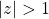. In fact

(2)such as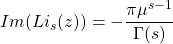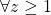, since we define

(3)and the equations

(4)(5)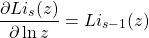(6)Duplication formula for the polylogarithm:

(7)Connection with the Kummer’s function can be established

(8)(9)and thus

(10)We also have

(11)Some extra values of (negative) integer polylogs that are rational functions or logarithms

(12)(13)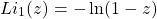(14)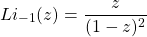(15)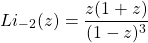(16)(17)And more generally, we have the general formulae

(18)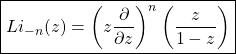(19)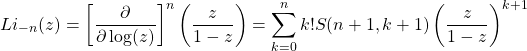and where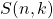are the Stirling numbers of the second kind.

(20)Furthermore,

(21)and where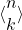are the eulerian numbers.

We write now some interesting values of the polylog you will love too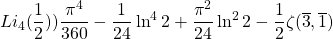and where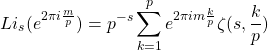with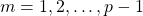.

The polylog and other functions can be also be related, as we have seen:

(22)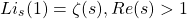(23)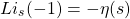(24)whereis the Dirichlet beta function. The complete Fermi-Dirac integral is also polylogarithmic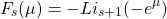The incomplete polylog is also interesting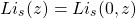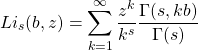and the incomplete gamma function is defined by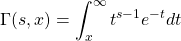(25)with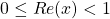,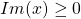,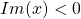. Moreover,

(26)There is also a formula called inversion formula

(27)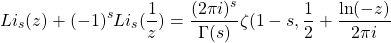and for(28)The expressionimplies that

(29)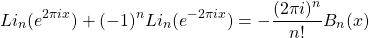withif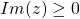andif. The following feynmanity (nullity) identity holds as well

(30)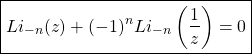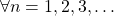and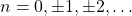(31)forand

(32)for. Polylogs and Clausen functions are related (we already saw this before)

(33)The inverse tangent integral is related to polylogs

(34)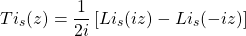(35)(36)(37)The Legendre chi function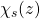is also related to polylogs

(38)The incomplete zeta function or Debye functions are polylogs as well

(39)(40)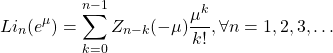(41)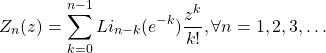(42)This last integral converges if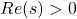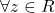and. It is the Bose-Einstein distribution!

(43)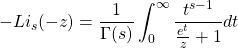For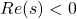and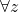exceptingandwe haveand

(44)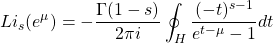with residue equal to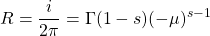See you in the next polylog post!

This site uses Akismet to reduce spam. Learn how your comment data is processed.